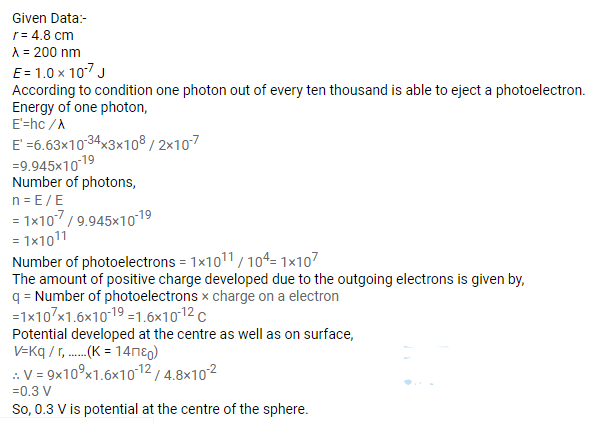# A silver ball of radius 4.8 cm is suspended by a thread in a vacuum chamber.

Question:

A silver ball of radius $4.8 \mathrm{~cm}$ is suspended by a thread in a vacuum chamber. Ultraviolet light of wavelength 200 nm is incident on the ball for some time during which light energy of $1.0 \times 10^{-7} \mathrm{~J}$ falls on the surface. Assuming that on average, one photon out of every ten thousand is able to eject a photoelectron, find the electric potential at the surface of the ball, assuming zero potential at infinity. What is the potential at the centre of the ball?

Solution: